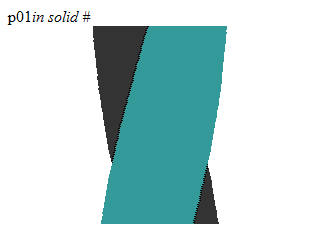# RUBBER IN SOLID #

183 wordsMathieu 'p01' Henri on January 6th, 2008

# Rubber in solid #

A crazy twister effect in 256bytes of DHTML## Source code

``p01<i id=d><script>setInterval('for(x=_="in solid #",E=Math,o=99,--O;o--;x+="<hr style=\'width:0;margin:auto;border-right:"+E.abs(q?p:P)+_+(9-q)*36+";border-left:"+E.abs(q?P:p)+_+(8+q)*36+"\'>")q=0>(p=E.cos(O+=22))*(P=E.sin(O));d.innerHTML=x',O=9)</script>``

Yes, pulling this one was not easy.

## How does it work

The twisting bar is rendered using `<hr>` elements whose border and color follow a curve. The borders are `solid` and sized fraction of `in` hence the subtitle of this intro.

This calculation `q=0>(p=E.cos(O+=22))(P=E.sin(O))` hits three birds. It computes and stores the length of both visible sides of the twisting bar in `p` and `P` and check and stores which one goes left by compairing `q=0>=p``P`.

With this three values the `border-right` and `border-left` of the `<hr>` element can style properly, setting the width to according to the visible sides of the bar and setting the color by casting `q` like this `(9-q)36` or `(8+q)``36`. Combined with the subtitle of this intro, this gives the colors `#288` and `#324`.